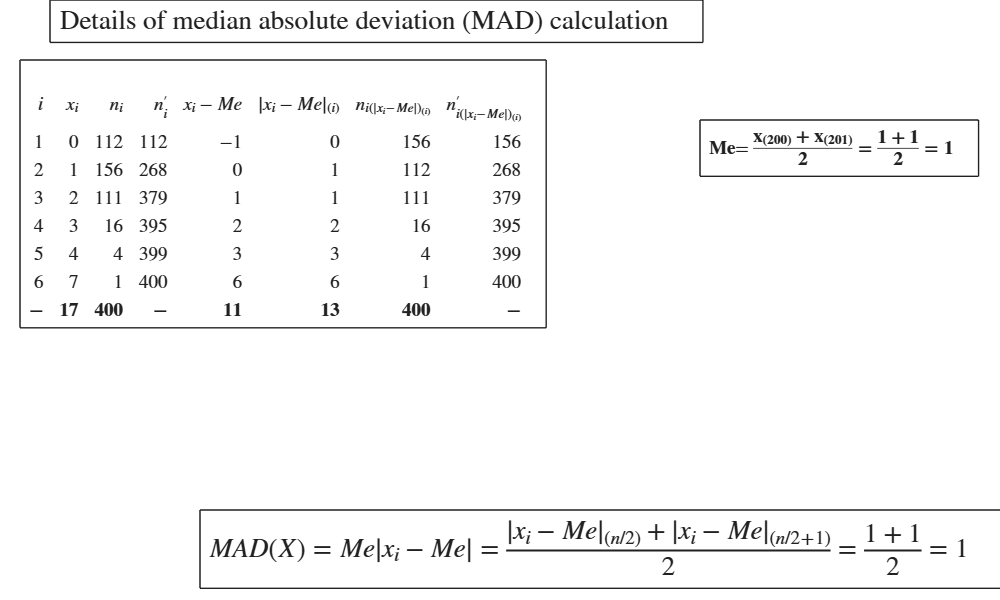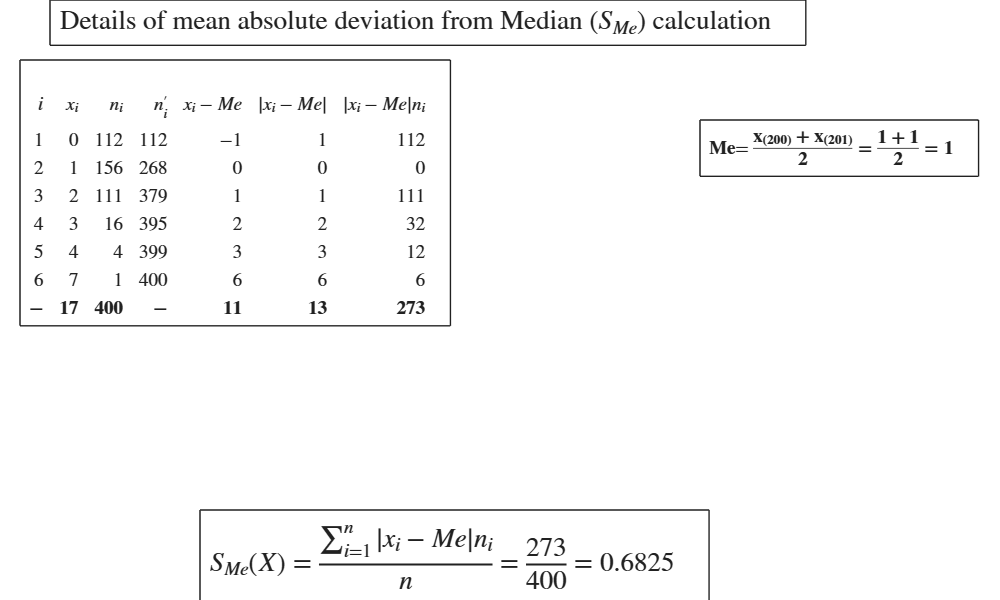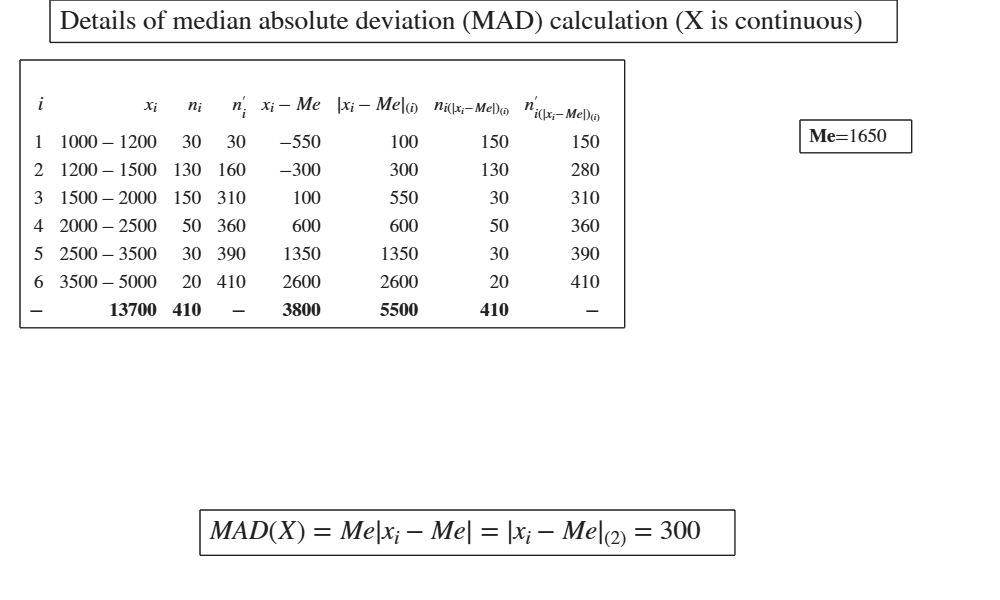# Documentation

GUImad shows the necessary calculations to obtain MAD, S_M or S_Me in a GUI.

## Syntax

• out=GUImad(x)example
• out=GUImad(x, flag)example
• out=GUImad(x, flag, w)example
• out=GUImad(x, flag, w,Name,Value)example

## Description

This routine shows all the intermediate necessary steps to compute the three following variability indexes: $MAD =Me(|x_{i} - Me|n_i).$ $S_{M} = \frac{\sum_{i=1}^{r}|x_{i}-M| n_i}{n}$ $S_{Me} = \frac{\sum_{i=1}^r |x_i-Me|n_i}{n}.$

 out =GUImad(x) Example of calculation of MAD.

 out =GUImad(x, flag) Example of calculation of SMe.

 out =GUImad(x, flag, w) Example of calculation of SM.

 out =GUImad(x, flag, w, Name, Value) MAD in a frequency distribution (discrete data).

## Examples

expand all

### Example of calculation of MAD.

MAD = median absolute deviation from median.

x=[98 105 85 110 102];
y=GUImad(x);

### Example of calculation of SMe.

SMe= mean absolute deviation from the median.

x=[98 105 85 110 102];
y=GUImad(x,2);

### Example of calculation of SM.

SM= mean absolute deviation from mean.

x=[98 105 85 110 102];
y=GUImad(x,0);

###MAD in a frequency distribution (discrete data).

MAD = median absolute deviation from median.

% Frequency distribution of the number of children in a sample of 400
% families. (See page 29 of [CMR]).
X=[0	112
1	156
2	111
3	16
4	4
7	1];
x=X(:,1);
freq=X(:,2);
flag=1;
GUImad(x,flag,freq);## Related Examples

expand all

###SM in a frequency distribution (discrete data).

SM= mean absolute deviation from mean.

% Frequency distribution of the number of children in a sample of 400
% families. (See page 29 of [CMR]).
X=[0	112
1	156
2	111
3	16
4	4
7	1];
x=X(:,1);
freq=X(:,2);
flag=0;
GUImad(x,flag,freq);###SMe in a frequency distribution (discrete data).

SMe= mean absolute deviation from the median Frequency distribution of the number of children in a sample of 400 families. (See page 29 of [CMR]).

X=[0	112
1	156
2	111
3	16
4	4
7	1];
x=X(:,1);
freq=X(:,2);
flag=2;
GUImad(x,flag,freq);###MAD in a frequency distribution (continuous data).

Conside the following distribution of employees of a large company according to monthly gross wages: retr= classes of retribution (Euros)

retr=[1000 1200; 1200 1500; 1500 2000; 2000 2500; 2500 3500; 3500 5000];
% freq= frequencies
freq=[30; 130; 150; 50; 30; 20];
% x = vector which contains the extremes of the classes
x=[retr(1,1);retr(:,2)];
flag=1;
% GUImad is called with input option 'DiscreteData',false
GUImad(x,flag,freq,'DiscreteData',false);### SMe in a frequency distribution (continuous data).

SMe= median absolute deviation from mean.

% Conside the following distribution of employees of a large company
% according to monthly gross wages:
% retr= classes of retribution (Euros)
retr=[1000 1200; 1200 1500; 1500 2000; 2000 2500; 2500 3500; 3500 5000];
% freq= frequencies
freq=[30; 130; 150; 50; 30; 20];
% x = vector which contains the extremes of the classes
x=[retr(1,1);retr(:,2)];
flag=2;
% GUImad is called with input option 'DiscreteData',false
GUImad(x,flag,freq,'DiscreteData',false);

## Input Arguments

### x — vector of numeric data. Vector.

Vector containing strictly numerical data. Note that if x is referred to a continuous variable (that is option DiscreteData is false) x(1) represents the lower extreme of the first class, and the other values of x contain the upper extremes of the other classes.

Data Types: double, ordered categorical

### flag — median or mean absolute deviation from median or mean absolute deviation from mean. Scalar.

If flag=1 (default), MAD is based on medians, i.e. median(abs(x-median(x)).

elseif flag=0, $S_M$ is computed (mean absolute deviation) i.e. mean(abs(x-mean(x)).

elseif flag=2, $S_{Me}$ is computed (mean absolute deviation from median) i.e. mean(abs(x-median(x)).

Example: 1 

Data Types: double

### w — weights. Vector.

Vector of the same length of x containing the frequencies (weights) associated to each value of x if x is discrete or vector with length (x)-1 if variable X is continuous. If w is not supplied, it is assumed that all observations have the same (relative) frequency (weight).

Example: 1:10 

Data Types: double DiscreteData : Discrete data or continuous data. Boolean. If DiscreteData is true (default), we assume that the data in x come from a discrete variable. If DiscreteData is false, we assume that the data in x come from a continuous variable. Example - 'DiscreteData',false Data Types - boolean

### Name-Value Pair Arguments

Specify optional comma-separated pairs of Name,Value arguments. Name is the argument name and Value is the corresponding value. Name must appear inside single quotes (' '). You can specify several name and value pair arguments in any order as  Name1,Value1,...,NameN,ValueN.

Example:  1 , 1:10 , 'DiscreteData',false 

### flag —median or mean absolute deviation from median or mean absolute deviation from mean.scalar.

If flag=1 (default), MAD is based on medians, i.e. median(abs(x-median(x)).

elseif flag=0, $S_M$ is computed (mean absolute deviation) i.e. mean(abs(x-mean(x)).

elseif flag=2, $S_{Me}$ is computed (mean absolute deviation from median) i.e. mean(abs(x-median(x)).

Example:  1 

Data Types: double

### w —weights.vector.

Vector of the same length of x containing the frequencies (weights) associated to each value of x if x is discrete or vector with length (x)-1 if variable X is continuous. If w is not supplied, it is assumed that all observations have the same (relative) frequency (weight).

Example:  1:10 

Data Types: double

### DiscreteData —Discrete data or continuous data.boolean.

If DiscreteData is true (default), we assume that the data in x come from a discrete variable.

If DiscreteData is false, we assume that the data in x come from a continuous variable.

Example:  'DiscreteData',false 

Data Types: boolean

## Output Arguments

### out —detailed output to compute the index. Table

Table with n+1 rows (where n is the length of x) containing what is shown in the GUI. Last row contains the totals.

Cerioli, A., Milioli, M.A., Riani, M. (2016), "Esercizi di statistica (Quinta edizione)". [CMR]